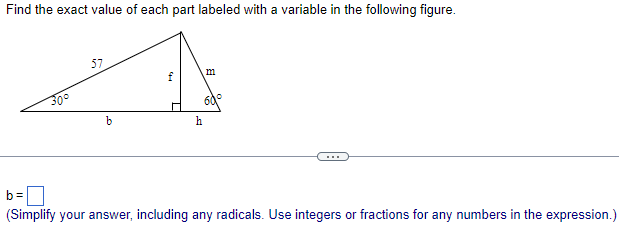Home / Expert Answers / Trigonometry / find-the-exact-value-of-each-part-labeled-with-a-variable-in-the-following-figure-b-simplify-yo-pa421

# (Solved): Find the exact value of each part labeled with a variable in the following figure. b= (Simplify yo ...Find the exact value of each part labeled with a variable in the following figure. (Simplify your answer, including any radicals. Use integers or fractions for any numbers in the expression.)

We have an Answer from Expert